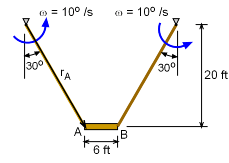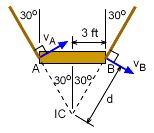Ch 5. Rigid Body General Motion Multimedia Engineering Dynamics Fixed Axis Rotation Plane Motion Velocities Zero Velocity Point Plane Motion Accelerations Multiple Gears Rot. Coord. Velocities Rot. Coord. Acceleration
 Chapter - Particle - 1. General Motion 2. Force & Accel. 3. Energy 4. Momentum - Rigid Body - 5. General Motion 6. Force & Accel. 7. Energy 8. Momentum 9. 3-D Motion 10. Vibrations Appendix Basic Math Units Basic Equations Sections Search eBooks Dynamics Fluids Math Mechanics Statics Thermodynamics Author(s): Kurt Gramoll ©Kurt GramollDYNAMICS - CASE STUDY SOLUTIONProblem DiagramIC Point Relative to Platform First, the velocity of points A and B can be easily determined by noting that the ropes rotate around a fixed point at the top of the building. Thus, the velocity at each point A and B on the work platform will be,      |rA| = 20/cos30 = 23.09 ft      ω = (10o/s)(π/180o) = 0.1745 rad/s      |vA| = |vB| = ω rA = (0.1745) (23.09) = 4.029 ft/s The direction of each velocity is perpendicular to the rotating rope as shown in the figure. The Instantaneous Center of Zero Velocity (IC) is found by drawing perpendicular lines from both velocities. The IC point represents the pivot or rotation point for both velocities. Therefore, the the angular velocity of bar AB is      v = ω d      4.03 = ω (3/sin30)      ω = 0.672 rad/s = 38.5o/s It is interesting to note that the platform will rotate nearly four times as fast as the rope. The bottom line is that Jim Knowitall, the new window washer, will not be safe and will probably be thrown off the platform.

Practice Homework and Test problems now available in the 'Eng Dynamics' mobile app
Includes over 400 problems with complete detailed solutions.
Available now at the Google Play Store and Apple App Store.